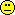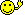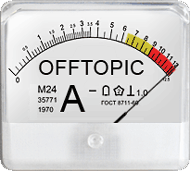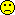mittyri
★★

Russia,
2019-03-16 14:41
(948 d 00:47 ago)

Posting: # 20038
Views: 4,565

## Handling BLOQ values (Fisher Info etc.) [Bioanalytics]

Dear All,

some time ago I was pleased to be invited on the session with Roger Jelliffe.
Some cites from one of the lectures:

Labs have been used to presenting a result only to generate a number, with a percent error, for evaluation by themselves or a clinician.
Labs have NOT been used to having their data FITTED using modern quantitative modeling methods which require one to evaluate credibility of a measurement correctly. That is the problem. Labs have been used to CV% only. CV% is simply not suitable for today’s modern quantitative modeling methods.
As measurement gets lower, CV% increases, and becomes infinite at zero. It fails. Always has, always will.
When CV% reaches some value, lab people think they must censor (withhold) results below this. Report “less than……”
They invent the LLOQ. LLOQ is their ILLUSION!
NO NEED for this!
Because SD, variance, and weight (1/var) are all finite throughout, all the way down to and including the zero blank.
Fisher Information quantifies the credibility of a lab measurement.
Fisher Information = 1/Var
So need to know, or have a good estimate, of the SD of every serum level.
Labs always get SD anyway, to get the CV%.
Then, Var = SD2
And Credibility = Fisher info = 1/Var.
Assay CV% versus correct weight, Fisher Info
Assume, for example, 10% assay CV
If conc = 10, SD = 1, var = 1, weight = 1
If conc = 20, SD = 2, var = 4, weight = ¼ - Aha!
So a constant linear % error (the assay CV%) is NOT the correct measure of the error!
Never was, never will be.
As conc approaches zero, CV% approaches infinity.
But assay SD, var, weight are always finite. Fisher info is the correct measure of assay precision.
Also, no need for any ILLUSORY LLOQ!

I've also seen some similar opinion on nmusers maillist (may be it was Nick Holford).

My question is are there any steps forward in that direction?
Even in analytical methodology (say QC samples): isn't possible to handle that values (as they are) for example for the mean evaluation for some level of QC sample?

PS: sorry, my bioanalytics skills are far away from acceptable levelKind regards,
Mittyri
Helmut
★★★Vienna, Austria,
2019-03-16 15:39
(947 d 23:50 ago)

@ mittyri
Posting: # 20039
Views: 4,086

## Handling BLOQ values (Fisher Info etc.)

Hi Mittyri,

» some time ago I was pleased to be invited on the session with Roger Jelliffe.

Congratulations! Roger is a guy of strong opinions.
When David Bourne’s PK/PD-List was still active, Roger regularly ranted about the LLOQ (examples). In PK modeling nobody simply ignores BLOQs. We still have no method dealing with “BQLs” in NCA (Martin).
Essentially Roger is absolutely right (speaking also from my background in analytical chemistry).BTW, on a similar note the best weighting scheme in calibration is 1/s2 – and not 1/x, 1/x2, 1/y, 1/y2. They are all arbitrary and lack any justification. OK, in between those lines of the EMA’s BMV guideline …

A relationship which can simply and adequately describe the response of the instrument with regard to the concentration of analyte should be applied.

… it seems that it is recommended not only to assess calibration functions themselves (chromatography: linear, quadratic, …; LBA: 4-, 5-parameter logistic, …) but also different weighting schemes (based on the back-calculated concentrations’ accuracy & precision). Rarely done.Of course, 1/s2 requires at least duplicates (even after rejecting a measurement). In our lab we had this procedure: Validate the method with 1/s2 and also the weighting scheme which gave the 2nd best outcome. Sometimes sponsors didn’t like triplicate standards (money, money). Then – if we ended up with a singlet – we switched to the other weighting scheme. Regulators didn’t like that (“subjects are not treated equally”).

» My question is are there any steps forward in that direction?

I strongly doubt it.

» Even in analytical methodology (say QC samples): isn't possible to handle that values (as they are) for example for the mean evaluation for some level of QC sample?

Not sure what you mean here.

Dif-tor heh smusma 🖖
Helmut SchützThe quality of responses received is directly proportional to the quality of the question asked. 🚮
Science Quotes
ElMaestro
★★★

Denmark,
2019-03-16 22:35
(947 d 16:54 ago)

@ Helmut
Posting: # 20041
Views: 4,025

## The smartest solution

I present to you the Philadelphia variation: The smartest and most empirical solution to a very, very small (and mainly theoretical?) problem.

Let us apply weights 1/C^z in such a fashion that the sum of relative absolute residuals is smallest. Idea borrowed from ISR.
And it may make good sense to look at the relative magnitude of residuals since this is what runs pass criteria are based on.

Therefore, here is something to play around with:
 Conc=c(1, 2, 4, 8, 20, 50, 100, 150, 200, 300) Ratio=c(0.5303, 0.1074, 0.2092, 0.4121, 0.9886, 2.3197, 5.0343, 7.7656, 10.2105, 14.9564) ObjF=function(z) {  w=1/(Conc^z)  M=lm(Ratio ~Conc, weight=w)  return(sum(abs(resid(M)/Conc))) } ##now let us find the value of z which gives the smallest sum of absolute relative residuals optimize(ObjF,  c(0, 10))

Note: the fit is not very good, r squared is rather low, but that is besides the point. You get my drift, I hope.

z then defines the weighting scheme which can be said to give the smallest overall amount of percent-wise prediction error on the calibration curve. Not a bad place to start.

You can modify the idea as you please, perhaps you want to define ObjF via the Ratio and not via the Conc, or perhaps you want to return another type of objective altogether. Various things that don't work include but aren't limited to abs sum of residuals, sum of residuals, and more.Thank me later.Pass or fail!
ElMaestro
Helmut
★★★Vienna, Austria,
2019-03-16 23:05
(947 d 16:24 ago)

@ ElMaestro
Posting: # 20042
Views: 4,016

## An old solution

Hi ElMaestro,

» […] The smartest and most empirical solution to a very, very small (and mainly theoretical?) problem.

Theoretical? Yes. Really relevant? Very, very rarely. Changing the weighting for all subjects to 1/y2 (we got two deficiency letters) altered the 90% CI in the second decimal place.

» Let us apply weights 1/C^z in such a fashion that ()

Congratulations for an obvious solution (aka, re-inventing the wheel).See what Ohlbe wrote here and there.

PS: Typo? Didn’t you want Ratio=0.05303?

Dif-tor heh smusma 🖖
Helmut SchützThe quality of responses received is directly proportional to the quality of the question asked. 🚮
Science Quotes
ElMaestro
★★★

Denmark,
2019-03-19 08:26
(945 d 07:03 ago)

@ Helmut
Posting: # 20049
Views: 3,764

## old, obvious?

Hi Hötzi,

» Congratulations for an obvious solution (aka, re-inventing the wheel).See what Ohlbe wrote here and there.

Is the proposal really old and is it really obvious?

I see there was in the past a proposal or two about 1/(Conc^z) where z is not an integer, but I am not sure if there was ever anyone who:
1. Defined an objective function which seeks to minimize a relevant measure of departure from predictability (that same predictability which maps into the criteria of guidelines).
2. Showed the existence of a minimum of that function.

Novelty here is not the existence of a funky value of z, but the way of finding it, the nature of it.

Pass or fail!
ElMaestro
Helmut
★★★Vienna, Austria,
2019-03-17 01:56
(947 d 13:32 ago)

@ ElMaestro
Posting: # 20043
Views: 4,033

## Example

Hi ElMaestro,

played with an example of a study I have on my desk. Chiral GC/MS, quadratic model, w=1/x2.

ObjF1 <- function(x) {   w <- 1/Conc^x   M <- lm(Ratio ~ Conc + I(Conc^2), weights=w)   return(sum(abs(resid(M)/Conc))) } ObjF2 <- function(x) {   w <- 1/Ratio^x   M <- lm(Ratio ~ Conc + I(Conc^2), weights=w)   return(sum(abs(resid(M)/Conc))) } IC <- function(m, n) {   return(list(AIC=signif(extractAIC(m, k=2),5),               BIC=signif(extractAIC(m, k=log(n))),5)) } Acc <- function(m, x, y) {   if (coef(m)[] == 0) stop("panic!")   if (coef(m)[] < 0 {     return(100*(-(coef(m)[]/2/coef(m)[] +                   sqrt((coef(m)[]/2/coef(m)[])^2-                        (coef(m)[]-y)/coef(m)[])))/x)   } else {     return(100*(-(coef(m)[]/2/coef(m)[] -                   sqrt((coef(m)[]/2/coef(m)[])^2-                        (coef(m)[]-y)/coef(m)[])))/x)   } } Conc  <- c(0.1, 0.1, 0.3, 0.3, 0.9, 0.9, 2, 2, 6, 6, 12, 12, 24, 24) Ratio <- c(0.022, 0.024, 0.073, 0.068, 0.193, 0.204, 0.438, 0.433,            1.374, 1.376, 2.762, 2.732, 5.616, 5.477) n     <- length(Conc) w.x1  <- 1/Conc w.x2  <- 1/Conc^2 x.opt <- optimize(ObjF1,  c(0, 10))$minimum w.xo <- 1/Conc^x.opt w.y1 <- 1/Ratio w.y2 <- 1/Ratio^2 y.opt <- optimize(ObjF2, c(0, 10))$minimum w.yo  <- 1/Ratio^x.opt dupl  <- sum(duplicated(Conc)) var   <- n/2 for (j in 1:dupl) {   var[j] <- var(c(Ratio[j], Ratio[j+1])) } w.var <- 1/rep(var, each=2) m.1   <- lm(Ratio ~ Conc + I(Conc^2)) m.2   <- lm(Ratio ~ Conc + I(Conc^2), weights=w.x1) m.3   <- lm(Ratio ~ Conc + I(Conc^2), weights=w.x2) m.4   <- lm(Ratio ~ Conc + I(Conc^2), weights=w.xo) m.5   <- lm(Ratio ~ Conc + I(Conc^2), weights=w.y1) m.6   <- lm(Ratio ~ Conc + I(Conc^2), weights=w.y2) m.7   <- lm(Ratio ~ Conc + I(Conc^2), weights=w.yo) m.8   <- lm(Ratio ~ Conc + I(Conc^2), weights=w.var) mods  <- c("w=1", "w=1/x", "w=1/x^2", "w=1/x^opt",            "w=1/y", "w=1/y^2", "w=1/y^opt", "w=1/sd.y^2") AIC   <- c(IC(m.1, n=n)$AIC, IC(m.2, n=n)$AIC, IC(m.3, n=n)$AIC, IC(m.4, n=n)$AIC,            IC(m.5, n=n)$AIC, IC(m.6, n=n)$AIC, IC(m.7, n=n)$AIC, IC(m.8, n=n)$AIC) BIC   <- c(IC(m.1, n=n)$BIC, IC(m.2, n=n)$BIC, IC(m.3, n=n)$BIC, IC(m.4, n=n)$BIC,            IC(m.5, n=n)$BIC, IC(m.6, n=n)$BIC, IC(m.7, n=n)$BIC, IC(m.8, n=n)$BIC) res1  <- data.frame(model=mods, exp=signif(c(0:2, x.opt, 1:2, y.opt, NA),5),                     AIC=signif(AIC,5), BIC=signif(BIC,5)) res2  <- data.frame(Conc=Conc,                     Acc(m=m.1, x=Conc, y=Ratio), Acc(m=m.2, x=Conc, y=Ratio),                     Acc(m=m.3, x=Conc, y=Ratio), Acc(m=m.4, x=Conc, y=Ratio),                     Acc(m=m.5, x=Conc, y=Ratio), Acc(m=m.6, x=Conc, y=Ratio),                     Acc(m=m.7, x=Conc, y=Ratio), Acc(m=m.8, x=Conc, y=Ratio)) names(res2) <- c("Conc", mods) cat("\nAkaike & Bayesian Information Critera (smaller is better)\n");print(res1);cat("\nAccuracy (%)\n");print(round(res2, 2), row.names=F)

I got:

 Akaike & Bayesian Information Critera (smaller is better)        model    exp      AIC      BIC 1        w=1 0.0000  -94.099  -92.181 2      w=1/x 1.0000 -127.480 -125.560 3    w=1/x^2 2.0000 -131.720 -129.800 4  w=1/x^opt 1.3355 -132.920 -131.010 5      w=1/y 1.0000 -106.670 -104.750 6    w=1/y^2 2.0000  -90.571  -88.654 7  w=1/y^opt 2.5220 -105.150 -103.230 8 w=1/sd.y^2     NA   62.387   64.304 Accuracy (%)  Conc    w=1  w=1/x w=1/x^2 w=1/x^opt  w=1/y w=1/y^2 w=1/y^opt w=1/sd.y^2   0.1 115.66  96.07   94.53     94.63  96.48   94.95     95.02      99.04   0.1 124.45 104.96  103.49    103.56 105.37  103.93    103.96     107.83   0.3 113.24 107.57  107.64    107.46 107.74  107.97    107.65     107.71   0.3 105.92 100.17  100.18    100.02 100.33  100.49    100.21     100.39   0.9  96.30  95.06   95.52     95.29  95.14   95.77     95.41      94.46   0.9 101.66 100.48  100.98    100.74 100.56  101.24    100.86      99.83   2.0  97.07  97.06   97.63     97.40  97.12   97.85     97.49      96.25   2.0  95.97  95.96   96.51     96.29  96.01   96.74     96.38      95.15   6.0 100.54 101.06  101.53    101.37 101.10  101.71    101.43     100.29   6.0 100.69 101.21  101.68    101.51 101.24  101.85    101.58     100.44  12.0 100.48 100.86  101.08    101.03 100.89  101.18    101.07     100.38  12.0  99.40  99.78  100.01     99.95  99.81  100.10     99.99      99.30  24.0 101.23 101.10  100.81    100.98 101.10  100.75    100.98     101.23  24.0  98.77  98.66   98.40     98.56  98.67   98.35     98.56      98.77

Hey, yours with w=1/x1.3355 is the winner! Duno why the ICs of 1/sy² are that bad. Coding error? The accuracy looks fine. Try a plot:

plot(Conc, Ratio, type="n", log="xy", las=1) points(Conc, Ratio, pch=21, cex=1.5, col="blue", bg="#CCCCFF80") curve(coef(m.4)[]+coef(m.4)[]*x+coef(m.4)[]*x^2, range(Conc),       lwd=2, col="darkgreen", add=TRUE) curve(coef(m.8)[]+coef(m.8)[]*x+coef(m.8)[]*x^2, range(Conc),       lwd=2, col="red", add=TRUE)

Dif-tor heh smusma 🖖
Helmut SchützThe quality of responses received is directly proportional to the quality of the question asked. 🚮
Science Quotes
mittyri
★★

Russia,
2019-03-18 22:20
(945 d 17:08 ago)

@ Helmut
Posting: # 20047
Views: 3,847

Hi Helmut,

Thank you so much for the detailed answer!

» » Even in analytical methodology (say QC samples): isn't possible to handle that values (as they are) for example for the mean evaluation for some level of QC sample?

» Not sure what you mean here.

Sorry for incomplete/incorrect question.
Say ADA, getting cut-off point (IV B FDA)
What would be a best tactics for cut-off point selection?
Say the data of healthy volunteers is {100, BLOQ, BLOQ}

Kind regards,
Mittyri
Helmut
★★★Vienna, Austria,
2019-03-19 01:34
(945 d 13:54 ago)

@ mittyri
Posting: # 20048
Views: 3,906

Hi mittyri,

» Say ADA, getting cut-off point (IV B FDA)
» What would be a best tactics for cut-off point selection?
» Say the data of healthy volunteers is {100, BLOQ, BLOQ}

No idea. That’s a minefield and beyond my competence. I prefer these Adas: #1, #2.Dif-tor heh smusma 🖖
Helmut SchützThe quality of responses received is directly proportional to the quality of the question asked. 🚮
Science Quotes
Ohlbe
★★★

France,
2019-03-17 14:32
(947 d 00:56 ago)

@ mittyri
Posting: # 20044
Views: 3,930

## Handling BLOQ values (Fisher Info etc.)

Dear Mittyri,

» Labs have NOT been used to having their data FITTED using modern quantitative modeling methods which require one to evaluate credibility of a measurement correctly. That is the problem. Labs have been used to CV% only. CV% is simply not suitable for today’s modern quantitative modeling methods.

» They invent the LLOQ. LLOQ is their ILLUSION!
» NO NEED for this!

» [So need to know, or have a good estimate, of the SD of every serum level.
» Labs always get SD anyway, to get the CV%.
» Then, Var = SD2

Mmmm. It is true that bioanalysts don't know how their data will be processed later. And they just don't care.

But PK scientists should also realise that the SD is not in any way a constant feature of a bioanalytical method. It can vary over time. From one run to the next (ion source getting dirty, sleepy or sloppy analyst etc.). And from one instrument to the next, should you have several involved in your study.

Not only that: precision is just one of the problems. Accuracy is another. Where does it come into Roger's picture ? The LLOQ is the lowest concentration you can measure with an acceptable precision and accuracy. Problem is, you can't really have a reliable estimate of accuracy below the LLOQ.

Question: where do you get your SD from ? Within-run ? Between-run ? Most importantly, from how many replicates ? In his response Helmut mentions SD calculated from 2 or 3 replicates. Sorry Helmut, but I would consider any such value as meaningless. To me, that would be the same thing as running a t-test or Chi-square on 5 values.

» My question is are there any steps forward in that direction?

Looking at the draft M10 guidance: nope.

Regards
Ohlbe
nobody
nothing

2019-03-18 08:14
(946 d 07:15 ago)

@ Ohlbe
Posting: # 20045
Views: 3,873

## Handling BLOQ values (Fisher Info etc.)

» In his response Helmut mentions SD calculated from 2 or 3 replicates. Sorry Helmut, but I would consider any such value as meaningless.

Exactly. Trash in trash out. SD is not erwartungstreu. Start with n=20+...

Kindest regards, nobody
Helmut
★★★Vienna, Austria,
2019-03-18 11:19
(946 d 04:10 ago)

@ nobody
Posting: # 20046
Views: 3,830

## Don’t weight by 1/s²

Hi nobody, Ohlbe and everybody,

» » In his response Helmut mentions SD calculated from 2 or 3 replicates. Sorry Helmut, but I would consider any such value as meaningless.
»
» Exactly. Trash in trash out. SD is not erwartungstreu. Start with n=20+...

I stand corrected – you are both right. Is a stupid idea.

Dif-tor heh smusma 🖖
Helmut SchützThe quality of responses received is directly proportional to the quality of the question asked. 🚮
Science QuotesIng. Helmut Schütz